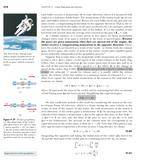Conservation of momentum

Homework Statement

A trolley of mass 200 kg moves with velocity of 10 m/s on a friction less track. A child of mass 20 kg runs on the trolley with a sped of 4 m/s relative to the trolley in the direction opposite to the trolley's motion and jumps out of the trolley. What is the final speed of the trolley?

Homework Equations

La of conservation of momentum

The Attempt at a Solution

My reference frame is moving with the velocity of the center of mass.
4*20=200*v
v=0.4
Therefore with respect to ground velocity of trolley=10.4 m/sec?

The confusion arises when I do it from the ground frame.
220*10=20(v-4)+ 200*v
solving to get v=10.36 m/s

Which of the two solutions is correct???

Doc Al
Mentor
My reference frame is moving with the velocity of the center of mass.
4*20=200*v
v=0.4
Therefore with respect to ground velocity of trolley=10.4 m/sec?
Careful. The speed of the child with respect to the trolley is 4m/s, not with respect to the center of mass.
The confusion arises when I do it from the ground frame.
220*10=20(v-4)+ 200*v
solving to get v=10.36 m/s
Much better.

Careful. The speed of the child with respect to the trolley is 4m/s, not with respect to the center of mass.

Much better.
But I thought the first one was correct. The reason being that the velocity of the center of mass and the initial velocity of the trolley was same.

Please have a look at the attached image. Its a page from a Physics Book written by Resnick Halliday & Walker. I have highlighted the points of doubt. The thing is that the calculations have been made in a similar fashion as my first method. Have a look.I am totally confused.

Doc Al
Mentor
The reason being that the velocity of the center of mass and the initial velocity of the trolley was same.
That's true, but note that the child's speed is given with respect to the trolley's final speed not the speed of the center of mass.

With the rocket equation things are different. With child and trolley we are dealing with finite (sizeable) changes in mass and speed, not just just tiny continuous deltas. Note that whether you measure the speed of the ejected fuel with respect to $v$ or $v + \Delta v$ doesn't really matter, since the $\Delta m \Delta v$ term would be dropped anyway.